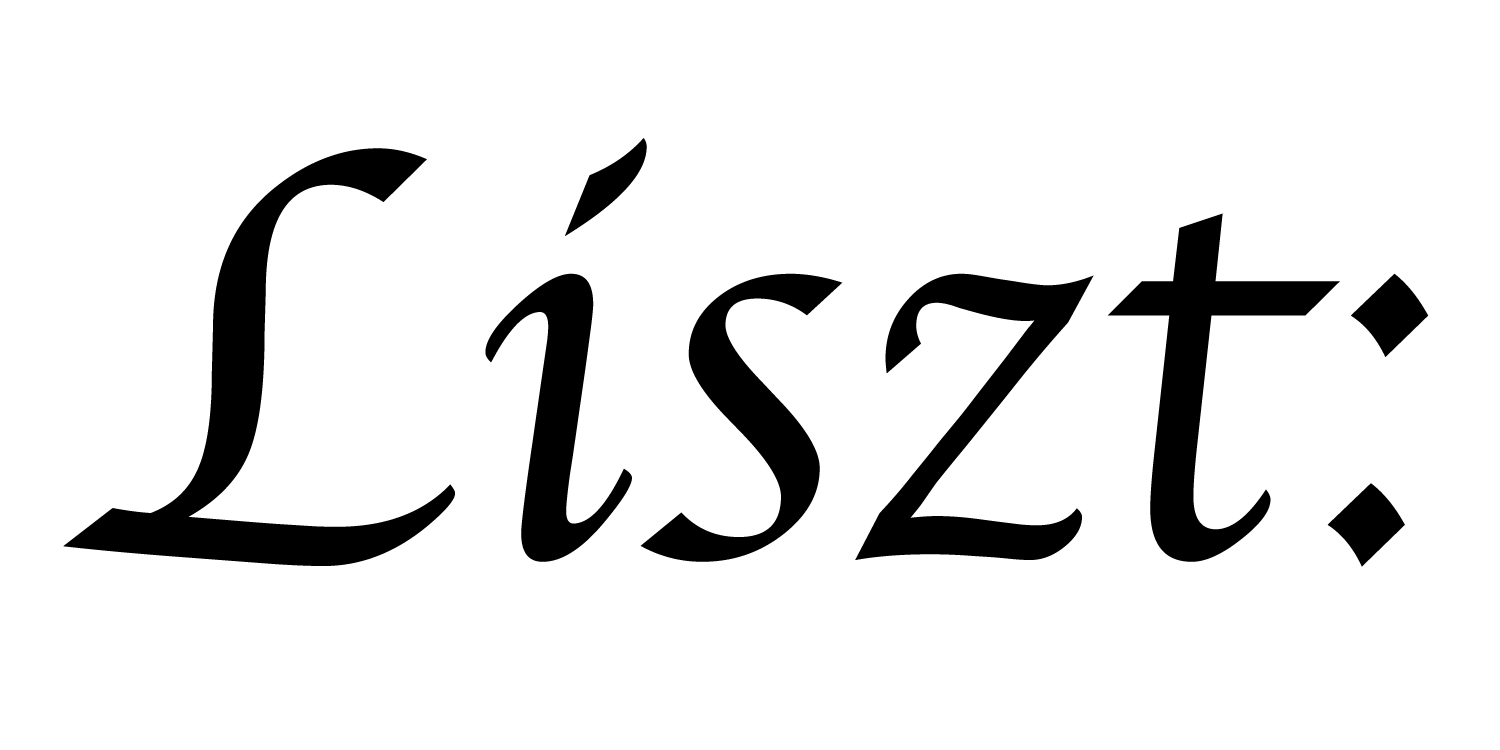# Ebb

is a programming language for writing physical simulations. Ebb programs are performance portable: they can be efficiently executed on both CPUs and GPUs. Ebb is embedded in the Lua programming language using Terra, which can itself be embedded in C/C++ programs as a library.

Ebb code looks like this:

``````import "ebb"
local L = require "ebblib"

local ioOff = require 'ebb.domains.ioOff'
local PN    = require 'ebb.lib.pathname'
local mesh  = ioOff.LoadTrimesh( PN.scriptdir() .. 'bunny.off' )

-- define globals and constants
local timestep   = L.Constant(L.double, 0.45)
local K          = L.Constant(L.double, 1.0)
local max_change = L.Global(L.double, 0.0)

-- define fields: temperature and change in temperature
if index == 0 then return 3000.0 else return 0.0 end
end)

-- functions executed for-each vertex
local ebb compute_diffusion ( v : mesh.vertices )
var count = 0.0
for nv in v.neighbors do
v.d_t += timestep * K * (nv.t - v.t)
count += 1.0
end
v.d_t = v.d_t / count
end

local ebb apply_update ( v : mesh.vertices )
v.t += v.d_t
max_change max= L.fabs(v.d_t)
v.d_t = 0.0
end

-- the simulation loop
for i = 1,300 do
if i % 30 == 0 then   max_change:set(0.0) end

mesh.vertices:foreach(compute_diffusion)
mesh.vertices:foreach(apply_update)

if i % 30 == 0 then   print('iter #'..i, max_change:get()) end
end
``````

Adding visualization routines, (in repository version) we can see the result of the above simulation.

Ebb was designed with a flexible data model that allows for encoding a range of different domains. As a non-exhaustive list, Ebb supports triangle meshes, grids, tetrahedral meshes, and particles. For example, here is a similar heat diffusion program written for a grid:

``````import 'ebb'
local L = require 'ebblib'

local GridLib   = require 'ebb.domains.grid'
local N = 40
local grid = GridLib.NewGrid2d {
size   = {N, N},
origin = {-N/2, -N/2},
width  = {N, N},
periodic_boundary = {true,true},
}

-- define constants, globals and fields
local timestep    = L.Constant(L.double, 0.45)
local conduction  = L.Constant(L.double, 1.0)
local max_diff    = L.Global(L.double, 0.0)

if x_idx == 4 and y_idx == 6 then return 1000 else return 0 end
end)

-- compute diffusion
local ebb update_temperature ( c : grid.cells )
var avg   = (1.0/4.0) * ( c(1,0).t + c(-1,0).t + c(0,1).t + c(0,-1).t )
var diff  = avg - c.t
c.new_t   = c.t + timestep * conduction * diff
end

-- measure statistic
local ebb measure_max_diff ( c : grid.cells )
var avg   = (1.0/4.0) * ( c(1,0).t + c(-1,0).t + c(0,1).t + c(0,-1).t )
var diff  = avg - c.t
max_diff  max= L.fabs(diff)
end

-- simulation loop
for i=1,360 do
grid.cells.interior:foreach(update_temperature)
grid.cells:Swap('t', 'new_t')

if i % 10 == 0 then -- measure statistics every 10 steps
max_diff:set(0)
grid.cells.interior:foreach(measure_max_diff)
print( 'iteration #'..tostring(i), 'max gradient: ', max_diff:get() )
end
end
``````

Again, we can visualize this simulation

Furthermore, domain libraries are user-authorable and can be coupled together in user code. For example, Ebb seamlessly supports coupling particles to a grid, or coupling the vertices of a mesh to a grid. By adding the following code to the preceding grid-based heat diffusion, we can set up a particle advection driven by the heat gradient.

``````-- create and initialize particle relation
local particles = L.NewRelation {
name = "particles",
size = N*N,
}

local particle_positions = {}
for yi=0,N-1 do
for xi=0,N-1 do
particle_positions[ xi*N + yi + 1 ] = { xi + 0.5,
yi + 0.5 }
end
end

-- establish link from particles to cells
particles:NewField('cell', grid.cells)
grid.locate_in_cells(particles, 'pos', 'cell')

local ebb wrap( x : L.double )
return L.fmod(x + 100*N, N)
end
local ebb advect_particle_position ( p : particles )
-- estimate heat gradient using a finite difference
var c   = p.cell
var dt  = { c(1,0).t - c(-1,0).t, c(0,1).t - c(0,-1).t }
-- and move the particle downwards along the gradient
var pos = p.pos - 0.1 * timestep * dt
-- wrap around the position...
p.pos = { wrap(pos), wrap(pos) }
end
``````

To advect the particles, we add two lines to the simulation loop:

``````for i=1,360 do
grid.cells:foreach(update_temperature)
grid.cells:Swap('t', 'new_t')

grid.locate_in_cells(particles, 'pos', 'cell')

if i % 10 == 0 then -- measure statistics every 10 steps
...
end
end
``````

The tutorials and code repository contain both simpler and more elaborate examples, explained in more detail.

## Liszt is …

A project at Stanford University to develop domain-specific languages for physical simulation. Liszt is focused on performance portability. Performance portable programs are programs that can be run efficiently on a variety of different parallel systems/platforms/architectures. (e.g. CPU, GPU, Multi-core, Clusters, Supercomputers)

Documentation and artifacts for the original Liszt language can be found online.

Ebb is the primary DSL for the Liszt project, with specialized DSLs for collision detection and other problems in the works.

### Ebb contributors

Gilbert Bernstein Chinmayee Shah Crystal Lemire Matthew Fisher Zach Devito Phil Levis Pat Hanrahan

a part of the Liszt project and PSAAP II center at Stanford University